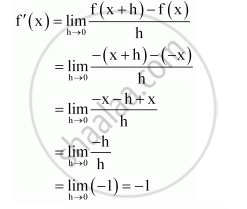CBSE (Commerce) Class 11CBSE
Share
Notifications

View all notifications
Books Shortlist
Your shortlist is empty

# Solution for Find the Derivative of the Following Functions from First Principle: −X - CBSE (Commerce) Class 11 - Mathematics

Login
Create free account

Forgot password?
ConceptIntroduction of Derivatives

#### Question

Find the derivative of the following functions from first principle:

−x

#### Solution

Let f(x) = –x. Accordingly,f(x + h)  = -(x +h)

By first principle,Is there an error in this question or solution?

#### APPEARS IN

NCERT Solution for Mathematics Textbook for Class 11 (2013 to Current)
Chapter 13: Limits and Derivatives
Q: 1.1 | Page no. 317
Solution Find the Derivative of the Following Functions from First Principle: −X Concept: Introduction of Derivatives.
S